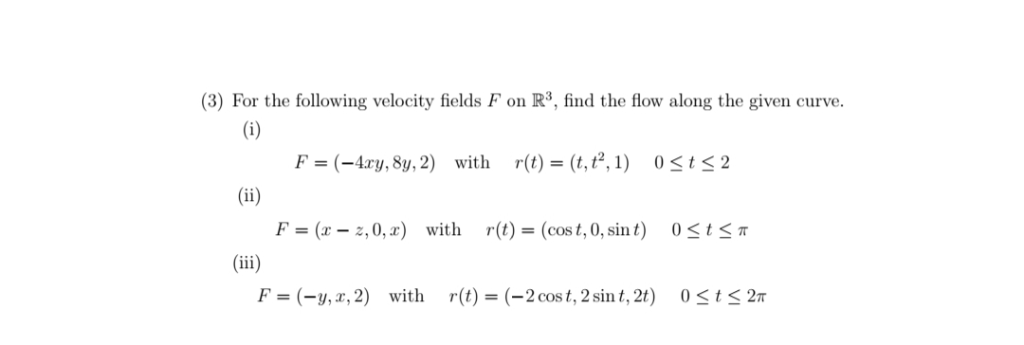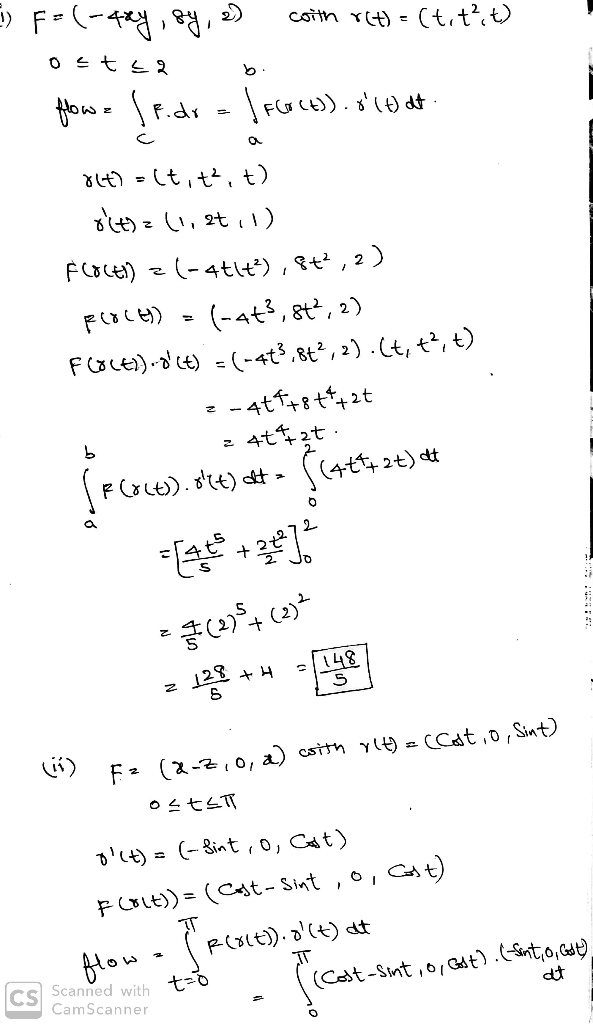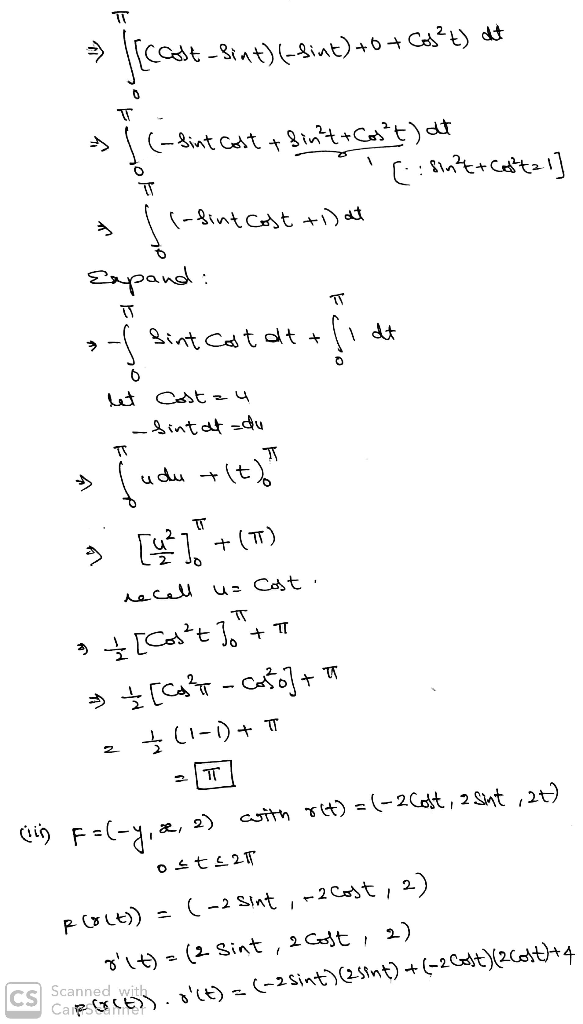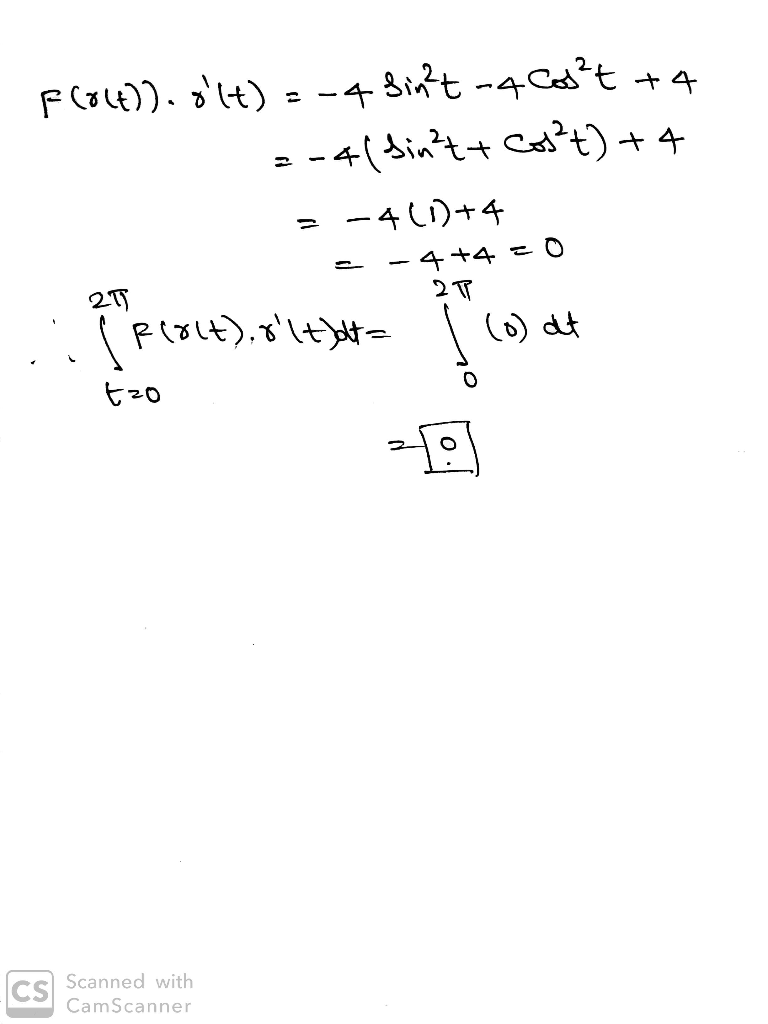Homework Help Question & Answers

(3) For the following velocity fields F on R3, find the flow along the given curve. r(t) = (t, t2, 1) F=(-4xy, 83, 2) with 0 2 t 1l F=(z-z, 0,2) r(t)-(cost, 0, sin t) with 0 t π F = (-y,2, 2) with r(...(3) For the following velocity fields F on R3, find the flow along the given curve. r(t) = (t, t2, 1) F=(-4xy, 83, 2) with 0 2 t 1l F=(z-z, 0,2) r(t)-(cost, 0, sin t) with 0 t π F = (-y,2, 2) with r(t) = (-2 cost, 2 sin t, 2t) 0Add Answer of: (3) For the following velocity fields F on R3, find the flow along the given curve. r(t) = (t, t2, 1) F=(-4xy, 83, 2) with 0 2 t 1l F=(z-z, 0,2) r(t)-(cost, 0, sin t) with 0 t π F = (-y,2, 2) with r(...
More Homework Help Questions Additional questions in this topic.

• The only ideas that can be used include: area ABCI-RA2(A+B+C-lpi), the Pythagorean theorem: Cos c cos a cos b. Vectors-dot product cross product, sin A-sin a/sin c; cos A-cos a sin b/sin c; spher...

Need Online Homework Help?

Get FREE EXPERT Answers
WITHIN MINUTES
Related Questions